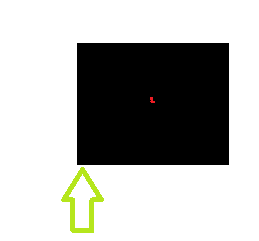# Constant Off-Center Propulsion (without gravity)

• B
Gabriel Mota
Hi,

How to calculate the position in time by applying a force, like a Thrust, not in the center of mass:I only know about the linear and angular formulas: Pos/rotation = 1/2 * F * Time^2

I have struggled finding topics about this maybe because i don't know the correct nomenclatures and maths.

#### Attachments

Homework Helper
Gold Member
2022 Award
First step is to be clear about what exactly is constant here. Is the direction and point of application of thrust constant relative to the rigid body, as would be the case for an engine within the body, or is the point of application fixed in the body but the direction fixed in the rest frame?

Gabriel Mota
First step is to be clear about what exactly is constant here. Is the direction and point of application of thrust constant relative to the rigid body, as would be the case for an engine within the body, or is the point of application fixed in the body but the direction fixed in the rest frame?

By constant i mean the same force is constantly being applied and as you mention, could be for example a spaceship with an engine.

Homework Helper
Gold Member
2022 Award

By constant i mean the same force is constantly being applied and as you mention, could be for example a spaceship with an engine.
You seem to have missed the crux of my question.
I understand that the force is to be of constant magnitude, but what about its direction? If it is from an engine rigidly fixed to the body then its direction changes as the body rotates; but if it is from an outside source then the direction may be constant in the inertial frame.

Gabriel Mota
You seem to have missed the crux of my question.
I understand that the force is to be of constant magnitude, but what about its direction? If it is from an engine rigidly fixed to the body then its direction changes as the body rotates; but if it is from an outside source then the direction may be constant in the inertial frame.

Yes, the engine would be fixed and rotates with the rigid body.

Homework Helper
Gold Member
2022 Award
Yes, the engine would be fixed and rotates with the rigid body.
Ok, so choose a coordinate system and define some variables.
In Cartesian, with x, y as the coordinates of the mass centre, you need the angle the body makes to the x axis, and an offset distance from the mass centre to the line of action of the thrust. Take the offset as being in the +x direction when the angle is zero.